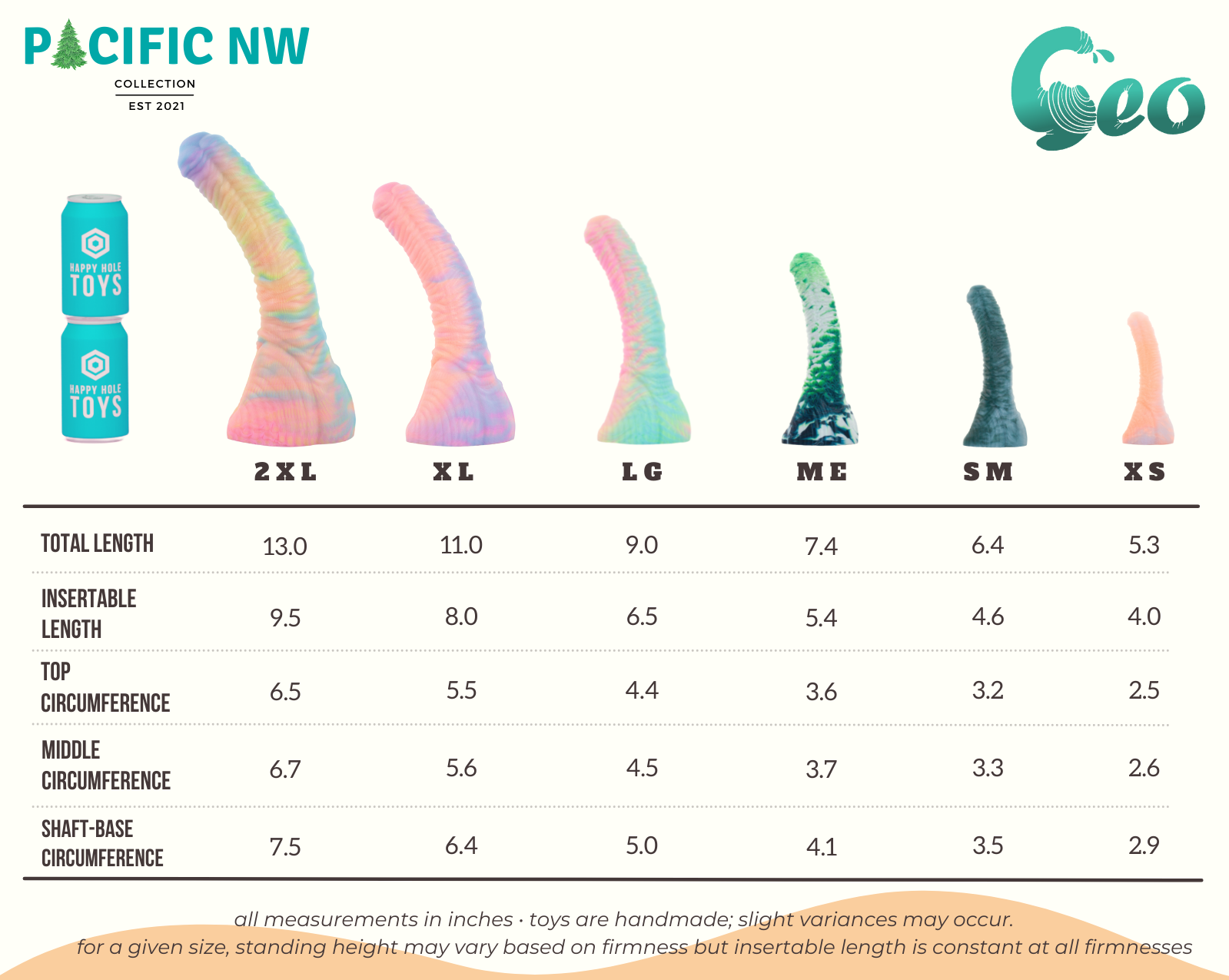# Geo Size Chart

Text-reader friendly Geo size information can be found after this image:OCR-friendly Geo size information:

• Geo XSmall: Total Length is 5.3 inches and the Insertable Length is 4.0 inches. The Top Circumference is 2.5 inches, the Middle Circumference is 2.6 inches, and the Shaft-Base Circumference is 2.9 inches
• Geo Small: Total Length is 6.4 inches and the Insertable Length is 4.6 inches. The Top Circumference is 3.2 inches, the Middle Circumference is 3.3 inches, and the Shaft-Base Circumference is 3.5 inches.
• Geo Medium: Total Length is 7.4 inches and the Insertable Length is 5.4 inches. The Top Circumference is 3.6 inches, the Middle Circumference is 3.7 inches, and the Shaft-Base Circumference is 4.1 inches.
• Geo Large: Total Length is 9.0 inches and the Insertable Length is 6.5 inches. The Top Circumference is 4.4 inches, the Middle Circumference is 4.5 inches, and the Shaft-Base Circumference is 5.0 inches.
• Geo XLarge: Total Length is 11.0 inches and the Insertable Length is 8.0 inches. The Top Circumference is 5.5 inches, the Middle Circumference is 5.6 inches, and the Shaft-Base Circumference is 6.4 inches.
• Geo XXLarge: Total Length is 13.0 inches and the Insertable Length is 9.5 inches. The Top Circumference is 6.5 inches, the Middle Circumference is 6.7 inches, and the Shaft-Base Circumference is 7.5 inches.